### Nscape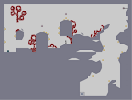Hover over the thumbnail for a full-size version.

Author diminishedD6 author:diminishedd6 editmode enter-respawn unrated 2010-07-28 2010-07-28 4 more votes required for a rating. \$Nscape#diminishedD6#enter-respawn#000000000000111111111110000000000001111111111100000000000011111111111000000?1100111111111111000000>1M001111111111110000000000011111111111100000000000B11111111111000000000000111111111110000000C111111111111111000000011111111111111110000000B1111111111111110000000000011111111111100000000000111111111111000000000001111111111110000C11100C111111111111000011110011111111111110000B111@0111E1111111110000000?11111P1111111110000000N111E1D1111111110000000>111P1111111A00000000000111P111111E000000000000B11P1E111M0000000000000CB1DE0B11@000000000000K1C1100C11A000000000003A0FJM00FQI00000000000CA000000000000000G00000I000000000000031110000000000000000000E111000000031H000GH00000>11000000011100C11111111110GO0H0C111@G11111111111|5^396,252!3^396,84!3^252,156!0^264,192!0^288,204!0^396,108!0^408,120!0^384,120!1^366,168!1^282,234!0^294,234!0^354,168!1^102,216!1^18,252!1^18,174!0^30,252!0^90,216!0^30,174!0^30,174!1^366,198!0^354,198!4^48,306,1!2^132,180,0.707106781186547,-0.707106781186547!2^492,204,0.707106781186547,-0.707106781186547!12^294,276!12^336,282!12^330,276!12^334,278!12^321,274!12^317,267!12^311,266!12^193,301!12^196,297!12^201,292!12^207,289!12^213,289!12^214,295!12^199,303!12^196,308!12^207,296!12^200,299!12^202,309!12^204,305!12^207,304!12^213,303!12^210,311!12^214,312!12^194,293!12^189,291!12^184,294!12^188,297!12^205,281!12^205,273!12^204,265!12^203,256!12^206,251!12^211,247!12^215,246!12^204,287!12^206,277!12^201,237!12^201,245!12^202,252!12^203,256!12^203,260!12^205,269!12^197,217!12^190,219!12^187,226!12^187,232!12^190,236!12^195,239!12^192,230!12^195,238!12^211,215!12^216,224!12^206,240!12^214,238!12^203,213!12^197,263!12^190,258!12^188,253!12^189,250!12^194,247!12^187,245!12^181,242!12^177,262!12^186,265!12^174,247!12^173,257!12^208,267!12^214,264!12^217,259!12^303,270!12^288,279!12^335,288!12^340,287!12^416,218!12^411,222!12^414,218!12^114,181!12^104,178!12^110,177!12^99,183!12^98,191!12^102,191!12^105,185!12^110,181!12^97,187!12^102,125!12^102,115!12^103,102!12^103,87!12^103,95!12^102,107!12^101,117!12^108,130!12^106,121!12^106,115!12^106,109!12^106,102!12^107,91!12^106,98!12^110,115!12^117,107!12^114,110!12^119,102!12^122,96!12^131,92!12^125,95!12^139,108!12^136,94!12^135,115!12^130,119!12^123,119!12^118,114!12^140,99!12^126,100!12^122,106!12^128,119!12^122,112!12^129,115!12^141,106!12^132,98!12^119,119!12^102,131!12^142,113!12^136,119!12^139,117!12^106,126!12^145,73!12^137,70!12^133,76!12^133,86!12^139,90!12^145,88!12^153,83!12^139,84!12^145,83!12^132,81!12^152,87!12^147,91!12^143,68!12^149,69!12^153,70!12^157,79!12^154,75!12^100,91!12^98,83!12^80,48!12^80,57!12^81,59!12^85,70!12^85,71!12^88,75!12^89,77!12^93,82!12^96,84!12^81,62!12^79,53!12^82,69!12^102,82!12^107,61!12^104,81!12^104,87!12^105,78!12^107,70!12^107,67!12^108,62!12^105,68!12^104,79!12^104,74!12^109,61!12^83,66!12^77,38!12^73,38!12^70,42!12^68,55!12^68,56!12^71,60!12^77,61!12^87,47!12^85,43!12^80,40!12^79,40!12^87,52!12^85,58!12^84,59!12^81,60!12^67,52!12^71,57!12^71,59!12^76,59!12^81,56!12^80,43!12^72,56!12^76,71!12^68,72!12^67,75!12^67,81!12^67,84!12^71,90!12^73,92!12^80,90!12^85,85!12^85,80!12^76,73!12^71,73!12^73,88!12^76,89!12^76,88!12^72,85!12^83,87!12^104,57!12^116,42!12^124,49!12^125,57!12^112,68!12^116,68!12^121,66!12^125,61!12^125,60!12^125,57!12^103,54!12^114,62!12^116,60!12^118,55!12^123,43!12^98,50!12^99,59!12^103,64!12^98,56!12^98,45!12^103,39!12^115,40!12^111,37!12^121,40!12^106,37!12^115,36!12^99,41!12^405,221!12^403,218!12^408,218!12^418,228!12^425,234!12^421,232!12^424,237!12^426,243!12^427,249!12^427,249!12^427,254!12^427,260!12^427,262!12^427,262!12^432,256!12^432,244!12^430,236!12^416,225!12^422,224!12^426,226!12^429,236!12^429,244!12^425,232!12^423,229!12^429,230!12^429,232!12^430,243!12^430,239!12^429,259!12^430,247!12^431,250!12^429,255!12^433,264!12^433,264!12^432,264!12^428,264!12^416,223!12^420,223!12^211,213!12^208,210!12^202,210!12^198,210!12^196,213!12^189,223!12^191,212!12^189,230!12^187,235!12^190,237!12^193,240!12^195,240!12^201,241!12^202,242!12^205,242!12^208,240!12^212,238!12^215,236!12^215,236!12^215,239!12^210,241!12^215,242!12^203,247!12^182,241!12^177,241!12^174,245!12^173,251!12^172,256!12^172,260!12^174,263!12^178,264!12^180,264!12^182,266!12^186,265!12^190,263!12^191,260!12^191,258!12^192,255!12^192,252!12^190,250!12^188,246!12^180,259!12^186,257!12^212,218!12^216,219!12^215,229!12^66,48!12^306,278!12^307,267!12^320,271!12^301,276!12^296,277!12^324,278!12^318,281!12^321,276!12^323,275!0^732,312!0^624,300!0^618,360!0^654,486!0^558,456!0^277,196!9^756,48,1,0,31,2,0,0,-1!10^742,49!11^492,564,492,564!0^744,0!0^756,156!0^624,276!0^708,252!0^708,348!12^450,136!12^448,133!12^446,131!12^443,130!12^440,131!12^439,132!12^453,145!12^453,151!12^447,159!12^442,165!12^441,165!12^437,165!12^435,164!12^433,163!12^432,160!12^450,158!12^451,139!12^451,156!12^446,181!12^445,186!12^444,186!12^444,191!12^447,192!12^458,183!12^450,181!12^447,182!12^455,179!12^451,195!12^457,193!12^459,185!12^458,188!12^436,170!12^432,188!12^432,186!12^434,175!12^434,179!12^433,182!12^433,193!12^433,198!12^433,203!12^433,205!12^437,216!12^434,212!12^433,209!12^441,193!12^440,193!12^436,195!12^435,197!12^433,200!12^447,165!12^433,126!12^423,128!12^427,126!12^427,124!12^428,124!12^433,129!12^435,155!12^441,158!12^449,188!12^444,151!12^439,154!12^569,185!12^568,188!12^585,202!12^587,204!12^578,204!12^571,215!12^578,192!12^579,190!12^582,183!12^582,184!12^581,188!12^571,217!12^596,188!12^590,188!12^585,188!12^578,193!12^599,185!12^600,187!12^606,171!12^602,183!12^566,195!12^576,204!12^567,198!12^568,202!12^571,205!12^603,180!12^604,177!12^573,200!12^570,208!12^576,198!12^569,209!12^565,212!12^563,217!12^563,221!12^552,207!12^551,208!12^549,212!12^548,218!12^552,224!12^557,227!12^557,228!12^556,230!12^611,153!12^614,153!12^620,158!12^622,165!12^621,169!12^620,170!12^615,153!12^617,154!12^620,160# play in edit mode and respawn with Enter instead of ~ + R

## Other maps by this author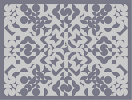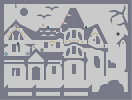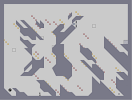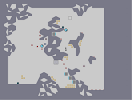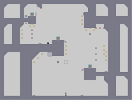Lucy in the Sky with Diamonds Enter...IF YOU DARE! Adderall & Cannabis A Face... I Have The Moon, You Have The Internet Avatar Portrait Contest